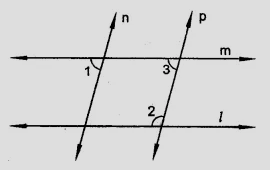"
">

# In the figure, if $l \parallel m, n \parallel p$ and $\angle 1 = 85^o$, find $\angle 2$."

Given:

$l \parallel m, n \parallel p$ and $\angle 1 = 85^o$.
To do:

We have to find $\angle 2$.

Solution:

$n \parallel p$

This implies,

$\angle 1 = \angle 3$               (Corresponding anlges are equal)

$\angle 3 =\angle 1 = 85^o$

$m \parallel l$

Therefore,

$\angle 3 + \angle 2 = 180^o (Co interior angles are supplementary)$85^o + \angle 2 = 180^o\angle 2 = 180^o - 85^o\angle 2 = 95^o$Hence,$\angle 2 = 95^o\$.

Updated on: 10-Oct-2022

34 Views